Updating search results...

# 83 Results

View
Selected filters:
• CCSS.Math.Content.6.RP.A.3Educational Use
Rating
0.0 stars

Bianca visits a bike shop and learns how bicycle gears work in this Cyberchase video segment.

Subject:
Mathematics
Material Type:
Lecture
Provider:
PBS LearningMedia
Provider Set:
PBS Learning Media: Multimedia Resources for the Classroom and Professional Development
Author:
U.S. Department of Education
WNET
09/09/2008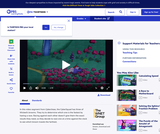Educational Use
Rating
0.0 stars

The CyberSquad tests which broom can travel the furthest in five seconds in this video from Cyberchase.

Subject:
Mathematics
Physics
Material Type:
Lecture
Provider:
PBS LearningMedia
Provider Set:
PBS Learning Media: Multimedia Resources for the Classroom and Professional Development
Author:
U.S. Department of Education
WNET
07/08/2008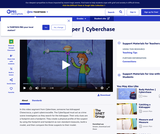Educational Use
Rating
0.0 stars

In this video segment from Cyberchase, the CyberSquad and Digit construct a physical profile of the person who kidnapped Choocroca, a giant cybercrocodile.

Subject:
Mathematics
Material Type:
Lecture
Provider:
PBS LearningMedia
Provider Set:
PBS Learning Media: Multimedia Resources for the Classroom and Professional Development
Author:
U.S. Department of Education
WNET
09/10/2008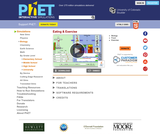Unrestricted Use
CC BY
Rating
0.0 stars

How many calories are in your favorite foods? How much exercise would you have to do to burn off these calories? What is the relationship between calories and weight? Explore these issues by choosing diet and exercise and keeping an eye on your weight.

Subject:
Health, Medicine and Nursing
Life Science
Anatomy/Physiology
Biology
Ecology
Forestry and Agriculture
Mathematics
Physics
Material Type:
Activity/Lab
Interactive
Simulation
Provider:
Provider Set:
PhET Interactive Simulations
Author:
Franny Benay
Kate Semsar
Kathy Perkins
Noah
Noah Podolefsky
Sam Reid
10/01/2008Only Sharing Permitted
CC BY-NC-ND
Rating
4.5 stars

This lesson unit is intended to help teachers assess how well students are able to visualize two-dimensional cross-sections of representations of three-dimensional objects. In particular, the lesson will help you identify and help students who have difficulties recognizing and drawing two-dimensional cross-sections at different points along a plane of a representation of a three-dimensional object.

Subject:
Geometry
Material Type:
Assessment
Lesson Plan
Provider:
Shell Center for Mathematical Education
Provider Set:
Mathematics Assessment Project (MAP)
04/26/2013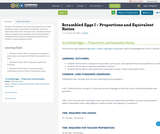Conditional Remix & Share Permitted
CC BY-NC-SA
Rating
0.0 stars

Students will analyze ratios and use proportions to solve problems using a cooperative, kinesthetic activity in which they will create “human ratios.” Students will use ratios to compare two quantities, then solve problems cooperatively by demonstrating how proportions are written to show equivalent ratios.

Subject:
Mathematics
Material Type:
Activity/Lab
Teaching/Learning Strategy
Author:
01/28/2016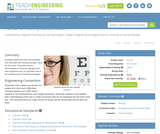Educational Use
Rating
4.0 stars

In this activity, students determine their own eyesight and calculate what a good average eyesight value for the class would be. Students learn about technologies to enhance eyesight and how engineers play an important role in the development of these technologies.

Subject:
Engineering
Material Type:
Activity/Lab
Provider:
TeachEngineering
Provider Set:
TeachEngineering
Author:
Denali Lander
Janet Yowell
Joe Freidrichsen
Malinda Schaefer Zarske
09/26/2008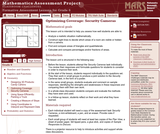Only Sharing Permitted
CC BY-NC-ND
Rating
0.0 stars

This lesson unit is intended to help sixth grade teachers assess how well students are able to: Analyze a realistic situation mathematically; construct sight lines to decide which areas of a room are visible or hidden from a camera; find and compare areas of triangles and quadrilaterals; and calculate and compare percentages and/or fractions of areas.

Subject:
Geometry
Ratios and Proportions
Material Type:
Assessment
Lesson Plan
Provider:
Shell Center for Mathematical Education
Provider Set:
Mathematics Assessment Project (MAP)
04/26/2013
Remix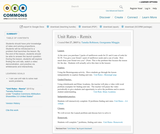Conditional Remix & Share Permitted
CC BY-SA
Rating
0.0 stars

Students should have prior knowledge of rates and solving proportions. Students will be introduced to a problem that launches the lesson. By the end of the lesson, students should be able to answer the launch question. During the lesson, students will explore finding the unit rate, watch a video demonstration, and practice via whiteboards and interactively.

Subject:
Mathematics
Material Type:
Lesson Plan
Author:
Tameka Robinson
Georgeanna Minggia
08/08/2019Conditional Remix & Share Permitted
CC BY-NC
Rating
0.0 stars

Math in Real Life (MiRL) supports the expansion of regional networks to create an environment of innovation in math teaching and learning.  The focus on applied mathematics supports the natural interconnectedness of math to other disciplines while infusing relevance for students.  MiRL supports a limited number of networked math learning communities that focus on developing and testing applied problems in mathematics.  The networks help math teachers refine innovative teaching strategies with the guidance of regional partners and the Oregon Department of Education.

Subject:
Mathematics
Material Type:
Homework/Assignment
Author:
Mark Freed
Tom Thompson
07/27/2020Conditional Remix & Share Permitted
CC BY-NC
Rating
0.0 stars

During this two-day lesson, students work with a partner to create and implement a problem-solving plan based on the mathematical concepts of rates, ratios, and proportionality. Students analyze the relationship between different-sized gummy bears to solve problems involving size and price.Key ConceptsThroughout this unit, students are encouraged to apply the mathematical concepts they have learned over the course of this year to new settings. Helping students develop and refine these problem solving skills:Creating a problem solving plan and implementing their plan systematicallyPersevering through challenging problems to find solutionsRecalling prior knowledge and applying that knowledge to new situationsMaking connections between previous learning and real-world problemsCommunicating their approaches with precision and articulating why their strategies and solutions are reasonableCreating efficacy and confidence in solving challenging problems in a real worldGoals and Learning ObjectivesCreate and implement a problem-solving plan.Organize and interpret data presented in a problem situation.Analyze the relationship between two variables.Use ratios.Write and solve proportions.Create rate tables to organize data and make predictionsUse multiple representations—including tables, graphs, and equations—to organize and communicate data.Articulate strategies, thought processes, and approaches to solving a problem and defend why the solution is reasonable.

Subject:
Ratios and Proportions
Material Type:
Lesson Plan
Provider:
Pearson
09/21/2015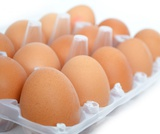Conditional Remix & Share Permitted
CC BY-NC
Rating
0.0 stars

In this lesson, students explore rate in the context of grocery shopping. Students use the unit price, or price per egg, to find the price of any number of eggs.Key ConceptsA unit price is a rate. The unit price tells the price of one unit of something (for example, one pound of cheese, one quart of milk, one box of paper clips, one package of cereal, and so on).The unit price can be found by dividing the price in dollars by the number of units.The unit price can be used to find the price of any quantity of something by multiplying the unit price by the quantity.Goals and Learning ObjectivesInvestigate rate as a unit price.Find a unit price by dividing the price in dollars by the number of units.Find the price of any quantity of something by multiplying that quantity by the unit price.

Subject:
Numbers and Operations
Material Type:
Lesson Plan
Provider:
Pearson
09/21/2015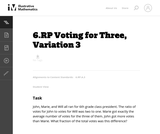Unrestricted Use
CC BY
Rating
0.0 stars

This is the last problem of seven in a series about ratios set in the context of a classroom election. Since the number of voters is not known, the problem is quite abstract and requires a deep understanding of ratios and their relationship to fractions.

Subject:
Mathematics
Material Type:
Activity/Lab
Provider:
Illustrative Mathematics
Provider Set:
Illustrative Mathematics
Author:
Illustrative Mathematics
05/01/2012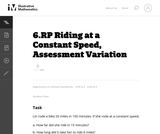Unrestricted Use
CC BY
Rating
0.0 stars

This is a task from the Illustrative Mathematics website that is one part of a complete illustration of the standard to which it is aligned. Each task has at least one solution and some commentary that addresses important asects of the task and its potential use. Here are the first few lines of the commentary for this task: Lin rode a bike 20 miles in 150 minutes. If she rode at a constant speed, How far did she ride in 15 minutes? How long did it take her to ride 6 miles?...

Subject:
Mathematics
Material Type:
Activity/Lab
Provider:
Illustrative Mathematics
Provider Set:
Illustrative Mathematics
Author:
Illustrative Mathematics
03/18/2013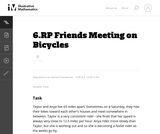Unrestricted Use
CC BY
Rating
0.0 stars

This series of 5 word problems lead up to the final problem. Most students should be able to answer the first two questions without too much difficulty. The decimal numbers may cause some students trouble, but if they make a drawing of the road that the girls are riding on, and their positions at the different times, it may help. The third question has a bit of a challenge in that students won't land on the exact meeting time by making a table with distance values every hour. The fourth question addresses a useful concept for problems involving objects moving at different speeds which may be new to sixth grade students.

Subject:
Mathematics
Material Type:
Activity/Lab
Provider:
Illustrative Mathematics
Provider Set:
Illustrative Mathematics
Author:
Illustrative Mathematics
05/01/2012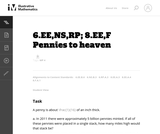Unrestricted Use
CC BY
Rating
0.0 stars

This is a task from the Illustrative Mathematics website that is one part of a complete illustration of the standard to which it is aligned. Each task has at least one solution and some commentary that addresses important asects of the task and its potential use. Here are the first few lines of the commentary for this task: A penny is about $\frac{1}{16}$ of an inch thick. In 2011 there were approximately 5 billion pennies minted. If all of these pennies were placed in a s...

Subject:
Mathematics
Material Type:
Activity/Lab
Provider:
Illustrative Mathematics
Provider Set:
Illustrative Mathematics
Author:
Illustrative Mathematics
03/17/2013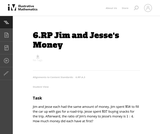Unrestricted Use
CC BY
Rating
0.0 stars

This word problem task involves ratios.

Subject:
Mathematics
Material Type:
Activity/Lab
Provider:
Illustrative Mathematics
Provider Set:
Illustrative Mathematics
Author:
Illustrative Mathematics
05/01/2012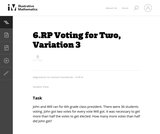Unrestricted Use
CC BY
Rating
0.0 stars

This problem, the third in a series of tasks set in the context of a class election, is more than just a problem of computing the number of votes each person receives. In fact, that isnŐt enough information to solve the problem. One must know how many votes it takes to make one half of the total number of votes. Although the numbers are easy to work with, there are enough steps and enough things to keep track of to lift the problem above routine.

Subject:
Mathematics
Material Type:
Activity/Lab
Provider:
Illustrative Mathematics
Provider Set:
Illustrative Mathematics
Author:
Illustrative Mathematics
05/01/2012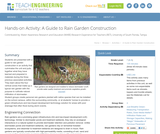Educational Use
Rating
0.0 stars

Students are presented with a guide to rain garden construction in an activity that culminates the unit and pulls together what they have learned and prepared in materials during the three previous associated activities. They learn about the four vertical zones that make up a typical rain garden with the purpose to cultivate natural infiltration of stormwater. Student groups create personal rain gardens planted with native species that can be installed on the school campus, within the surrounding community, or at students' homes to provide a green infrastructure and low-impact development technology solution for areas with poor drainage that often flood during storm events.

Subject:
Engineering
Hydrology
Material Type:
Activity/Lab
Provider:
TeachEngineering
Provider Set:
TeachEngineering
Author:
Brigith Soto
Jennifer Butler
Krysta Porteus
Maya Trotz
Ryan Locicero
William Zeman
09/18/2014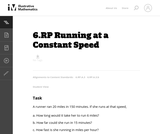Unrestricted Use
CC BY
Rating
0.0 stars

In this task students use ratio and rate reasoning to solve the real-world problem of a runners rate and distance.

Subject:
Mathematics
Material Type:
Activity/Lab
Provider:
Illustrative Mathematics
Provider Set:
Illustrative Mathematics
Author:
Illustrative Mathematics
05/01/2012Conditional Remix & Share Permitted
CC BY-NC
Rating
0.0 stars

Students use informal methods of their own choosing to find percents of randomly generated monetary values.Key ConceptsMany approaches work for solving percent problems. This lesson focuses on experimenting with a range of approaches and understanding why and how multiple approaches yield correct results.Goals and Learning ObjectivesFind a percent of a given quantity.Find a quantity given a part and the percent that part is of the whole.Use percents in money calculations.

Subject:
Ratios and Proportions
Material Type:
Lesson Plan
Provider:
Pearson
09/21/2015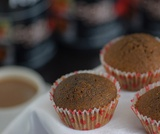Conditional Remix & Share Permitted
CC BY-NC
Rating
0.0 stars

Students focus on interpreting, creating, and using ratio tables to solve problems. They also relate ratio tables to graphs as two ways of representing a relationship between quantities.Key ConceptsRatio tables and graphs are two ways of representing relationships between variable quantities. The values shown in a ratio table give possible pairs of values for the quantities represented and define ordered pairs of coordinates of points on the graph representing the relationship. The additive and multiplicative structure of each representation can be connected, as shown: Goals and Learning ObjectivesComplete ratio tables.Use ratio tables to compare ratios and solve problems.Plot values from a ratio table on a graph.Understand the connection between the structure of ratio tables and graphs.

Subject:
Ratios and Proportions
Material Type:
Lesson Plan
Provider:
Pearson
09/21/2015Conditional Remix & Share Permitted
CC BY-NC
Rating
0.0 stars

Students use double number lines to model relationships and to solve ratio problems.Key ConceptsDouble number line diagrams are useful for visualizing ratio relationships between two quantities. They are best used when the quantities have different units. (The unit rate appears paired with 1.) Double number line diagrams help students more easily “see” that there are many equivalent forms of the same ratio.Goals and Learning ObjectivesUnderstand double number line diagrams as a way to visually compare two quantities.Use double number line diagrams to solve ratio problems.

Subject:
Ratios and Proportions
Material Type:
Lesson Plan
Provider:
Pearson
09/21/2015Conditional Remix & Share Permitted
CC BY-NC
Rating
0.0 stars

Subject:
Mathematics
Material Type:
Full Course
Provider:
Pearson
10/06/2016Rating
0.0 stars

Equations and Inequalities

Type of Unit: Concept

Prior Knowledge

Students should be able to:

Add, subtract, multiply, and divide with whole numbers, fractions, and decimals.
Use the symbols <, >, and =.
Evaluate expressions for specific values of their variables.
Identify when two expressions are equivalent.
Simplify expressions using the distributive property and by combining like terms.
Use ratio and rate reasoning to solve real-world problems.
Order rational numbers.
Represent rational numbers on a number line.

Lesson Flow

In the exploratory lesson, students use a balance scale to find a counterfeit coin that weighs less than the genuine coins. Then continuing with a balance scale, students write mathematical equations and inequalities, identify numbers that are, or are not, solutions to an equation or an inequality, and learn how to use the addition and multiplication properties of equality to solve equations. Students then learn how to use equations to solve word problems, including word problems that can be solved by writing a proportion. Finally, students connect inequalities and their graphs to real-world situations.

Subject:
Mathematics
Algebra
Material Type:
Unit of Study
Provider:
Pearson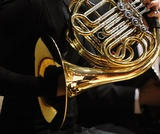Rating
0.0 stars

Rate

Type of Unit: Concept

Prior Knowledge

Students should be able to:

Solve problems involving all four operations with rational numbers.
Understand quantity as a number used with a unit of measurement.
Solve problems involving quantities such as distances, intervals of time, liquid volumes, masses of objects, and money, and with the units of measurement for these quantities.
Understand that a ratio is a comparison of two quantities.
Write ratios for problem situations.
Make and interpret tables, graphs, and diagrams.
Write and solve equations to represent problem situations.

Lesson Flow

In this unit, students will explore the concept of rate in a variety of contexts: beats per minute, unit prices, fuel efficiency of a car, population density, speed, and conversion factors. Students will write and refine their own definition for rate and then use it to recognize rates in different situations. Students will learn that every rate is paired with an inverse rate that is a measure of the same relationship. Students will figure out the logic of how units are used with rates. Then students will represent quantitative relationships involving rates, using tables, graphs, double number lines, and formulas, and they will see how to create one such representation when given another.

Subject:
Mathematics
Algebra
Material Type:
Unit of Study
Provider:
PearsonConditional Remix & Share Permitted
CC BY-NC
Rating
0.0 stars

During this two-day lesson, students work with a partner to create and implement a problem-solving plan based on the mathematical concepts of rates, ratios, and proportionality. Students analyze the relationship between different-sized gummy bears to solve problems involving size and price.Key ConceptsThroughout this unit, students are encouraged to apply the mathematical concepts they have learned over the course of this year to new settings. Help students develop and refine these problem-solving skills:Creating a problem solving plan and implementing their plan systematicallyPersevering through challenging problems to find solutionsRecalling prior knowledge and applying that knowledge to new situationsMaking connections between previous learning and real-world problemsCommunicating their approaches with precision and articulating why their strategies and solutions are reasonableCreating efficacy and confidence in solving challenging problems in a real worldGoals and Learning ObjectivesCreate and implement a problem-solving plan.Organize and interpret data presented in a problem situation.Analyze the relationship between two variables.Use ratios.Write and solve proportions.Create rate tables to organize data and make predictions.Use multiple representations—including tables, graphs, and equations—to organize and communicate data.Articulate strategies, thought processes, and approaches to solving a problem, and defend why the solution is reasonable.

Subject:
Ratios and Proportions
Material Type:
Lesson Plan
Provider:
Pearson
09/21/2015Conditional Remix & Share Permitted
CC BY-NC
Rating
0.0 stars

This lesson introduces the concept of a glide ratio and encourages students to use appropriate tools strategically (Mathematical Practice 5). Students use tape diagrams, double number lines, ratio tables, graphs, and equations to represent glide ratios.Key ConceptsA glide ratio for an object or an organism in flight is the ratio of forward distance to vertical distance (in the absence of power and wind). For a given object or organism that glides, this ratio has a constant value and is treated as a feature of the object or organism.Goals and Learning ObjectivesUnderstand the concept of a glide ratio.Make connections within and between different ways of representing ratios.

Subject:
Ratios and Proportions
Material Type:
Lesson Plan
Provider:
Pearson
09/21/2015Conditional Remix & Share Permitted
CC BY-NC
Rating
0.0 stars

Students watch a video in which a double number line is used to solve a problem about getting the right amount of protein mix. Using the double number line is an example of modeling with mathematics, which is Mathematical Practice 4.Key ConceptsA double number line shows corresponding values for two variable quantities with a constant ratio between them. Each pair of tick marks that go together shows a ratio equivalent to all of the other ratios between corresponding tick marks.Goals and Learning ObjectivesWatch an example of students using mathematics to model a relationship between quantities (MP4).Use a double number line to solve a problem.Use a double number line to deepen understanding of equivalence in the context of a relationship between quantities with a constant ratio.SWD: Some students with disabilities will benefit from a preview of the goals in each lesson. Students can highlight the critical features and/or concepts and will help them to pay close attention to salient information.

Subject:
Ratios and Proportions
Material Type:
Lesson Plan
Provider:
Pearson
09/21/2015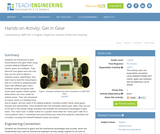Educational Use
Rating
0.0 stars

Students are introduced to gear transmissions and gear ratios using LEGO MINDSTORMS(TM) NXT robots, gears and software. They discover how gears work and how they can be used to adjust a vehicle's power. Specifically, they learn how to build the transmission part of a vehicle by designing gear trains with different gear ratios. Students quickly recognize that some tasks require vehicle speed while others are more suited for vehicle power. They are introduced to torque, which is a twisting force, and to speed the two traits of all rotating engines, including mobile robots using gears, bicycles and automobiles. Once students learn the principles behind gear ratios, they are put to the test in two simple design activities that illustrate the mechanical advantages of gear ratios. The "robot race" is better suited for a quicker robot while the "robot push" calls for a more powerful robot. A worksheet and post-activity quiz verify that students understand the concepts, including the tradeoff between torque and speed.

Subject:
Engineering
Material Type:
Activity/Lab
Provider:
TeachEngineering
Provider Set:
TeachEngineering
Author:
Michael Hernandez
Violet Mwaffo
09/18/2014Conditional Remix & Share Permitted
CC BY-NC
Rating
0.0 stars

Lesson OverviewStudents practice solving equations using either the addition or the multiplication property of equality.Key ConceptsStudents will solve equations of the form x + p = q using the addition property of equality.They will solve equations of the form px = q using the multiplication property of equality.They will need to look at the variable and decide what operation must be performed on both sides of the equation in order to isolate the variable on one side of the equation.If a number has been added to the variable, they will subtract that number from both sides of the equation. If a number has been subtracted from the variable, they will add that number to both sides of the equation. If the variable has been multiplied by a number, students will either divide both sides of the equation by that number or multiply by the reciprocal of that number. If the variable has been divided by a number, students will multiply by that number. Students will see how this can be applied to solving a proportion such as xc=ab.Goals and Learning ObjectivesPractice solving equations using either the addition or the multiplication property of equality.Distinguish between equations that can be solved using the addition property of equality from equations that can be solved using the multiplication property of equality.Solve a proportion by solving an equation.

Subject:
Algebra
Material Type:
Lesson Plan
Provider:
Pearson
09/21/2015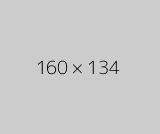Conditional Remix & Share Permitted
CC BY-NC
Rating
0.0 stars

Students watch a video in which two students discuss the problem of how to compare fuel efficiency. Students then analyze the work of the two students as they use rates to determine fuel efficiency in two different ways.Key ConceptsFuel efficiency is a rate. Fuel efficiency can be expressed in miles per gallon (mpg). This rate is useful for determining how far a vehicle can travel using any number of gallons of gas. Fuel efficiency can also be expressed in gallons per mile (gpm). This rate is useful for determining how many gallons of gas a vehicle uses to travel any number of miles.The rates miles per gallon and gallons per mile are inverse rates—they both describe the same relationship. For example, the rates 20 miles per gallon and 0.05 gallon per mile both describe the relationship between 300 miles and 15 gallons. The greater the rate in miles per gallon, the better the fuel efficiency. The smaller the rate in gallons per mile, the better the fuel efficiency.SWD: Some students with disabilities will benefit from a preview of the goals in each lesson. This will help to highlight for students the critical features and/or concepts and will help them to pay close attention to salient information.Goals and Learning ObjectivesExplore rate in the context of fuel efficiency.Express fuel efficiency as the rate miles per gallon (mpg) and as its inverse, gallons per mile (gpm).Use the rate miles per gallon to find the number of miles a car can travel on a number of gallons of gas.Use the rate gallons per mile to find the number of gallons of gas used for a number of miles driven.

Subject:
Algebra
Material Type:
Lesson Plan
Provider:
Pearson
09/21/2015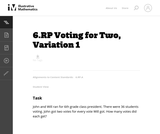Unrestricted Use
CC BY
Rating
0.0 stars

This is the first and most basic problem in a series of seven problems, all set in the context of a classroom election. Every problem requires students to understand what ratios are and apply them in a context. The problems build in complexity and can be used to highlight the multiple ways that one can reason about a context involving ratios.

Subject:
Mathematics
Material Type:
Activity/Lab
Provider:
Illustrative Mathematics
Provider Set:
Illustrative Mathematics
Author:
Illustrative Mathematics
05/01/2012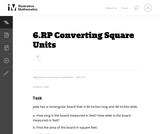Unrestricted Use
CC BY
Rating
0.0 stars

This task provides an opportunity to work on the Standard for Mathematical Practice 3 Construct Viable Arguments and Critique the Reasoning of Others.

Subject:
Mathematics
Material Type:
Activity/Lab
Provider:
Illustrative Mathematics
Provider Set:
Illustrative Mathematics
Author:
Illustrative Mathematics
05/01/2012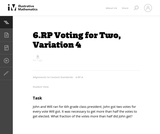Unrestricted Use
CC BY
Rating
0.0 stars

This is the fourth in a series of tasks about ratios set in the context of a classroom election. What makes this problem interesting is that the number of voters is not given. This information isnŐt necessary, but at first glance some students may believe it is.

Subject:
Mathematics
Material Type:
Activity/Lab
Provider:
Illustrative Mathematics
Provider Set:
Illustrative Mathematics
Author:
Illustrative Mathematics
05/01/2012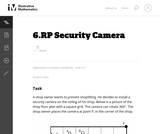Unrestricted Use
CC BY
Rating
0.0 stars

In this task students use ratio and rate reasoning to solve the real-world problem of placing a security camara in a shop.

Subject:
Mathematics
Material Type:
Activity/Lab
Provider:
Illustrative Mathematics
Provider Set:
Illustrative Mathematics
Author:
Illustrative Mathematics
05/01/2012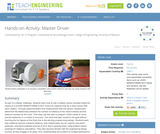Educational Use
Rating
0.0 stars

As part of a design challenge, students learn how to use a rotation sensor (located inside the casing of a LEGO® MINDSTORMS ® NXT motor) to measure how far a robot moves with each rotation. Through experimentation and measurement with the sensor, student pairs determine the relationship between the number of rotations of the robot's wheels and the distance traveled by the robot. Then they use this ratio to program LEGO robots to move precise distances in a contest of accuracy. The robot that gets closest to the goal without touching the toy figures at the finish line is the winning programming design. Students learn how rotational sensors measure distance, how mathematics can be used for real-world purposes, and about potential sources of error due to gearing when using rotation sensor readings for distance calculations. They also become familiar with the engineering design process as they engage in its steps, from understanding the problem to multiple test/improve iterations to successful design.

Subject:
Engineering
Electronic Technology
Material Type:
Activity/Lab
Provider:
TeachEngineering
Provider Set:
TeachEngineering
Author:
Nishant Sinha
Pranit Samarth
Satish S. Nair
09/18/2014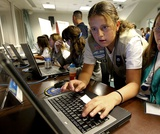Conditional Remix & Share Permitted
CC BY-NC
Rating
0.0 stars

Students focus on interpreting, creating, and using ratio tables to solve problems.Key ConceptsA ratio table shows pairs of corresponding values, with an equivalent ratio between each pair. Ratio tables have both an additive and a multiplicative structure:Goals and Learning ObjectivesComplete ratio tables.Use ratio tables to solve problems.

Subject:
Ratios and Proportions
Material Type:
Lesson Plan
Provider:
Pearson
09/21/2015Conditional Remix & Share Permitted
CC BY-NC
Rating
0.0 stars

Students work with a set of cards showing different ways of expressing ratios numerically. They group the cards showing equivalent ratios and then order the groups from least to greatest value.Key ConceptsIt can be hard to compare the values of ratios represented in different forms (e.g., a:b, decimal, fraction, a to b). Simplifying ratios makes it easier to compare and order their values.Goals and Learning ObjectivesIdentify ratios that are equivalent but expressed differently.Simplify ratios in order to group and order cards efficiently and successfully.

Subject:
Ratios and Proportions
Material Type:
Lesson Plan
Provider:
Pearson
09/21/2015Conditional Remix & Share Permitted
CC BY-NC
Rating
0.0 stars

Students create and implement a problem-solving plan to solve another problem involving the relationship between the sound of thunder and the distance of the lightning.Key ConceptsThroughout this unit, students are encouraged to apply the mathematical concepts they have learned over the course of this year to new settings. Help students develop and refine these problem-solving skills:Creating a problem-solving plan and implementing their plan systematicallyPersevering through challenging problems to find solutionsRecalling prior knowledge and applying that knowledge to new situationsMaking connections between previous learning and real-world problemsCommunicating their approaches with precision and articulating why their strategies and solutions are reasonableCreating efficacy and confidence in solving challenging problems in a real worldGoals and Learning ObjectivesCreate and implement a problem-solving plan.Organize and interpret data presented in a problem situation.Analyze the relationship between two variables.Create a rate table to organize data and make predictions.Apply the relationship between the variables to write a mathematical formula and use the formula to solve problems.Create a graph to display proportional relationships and use this graph to make predictions.Articulate strategies, thought processes, and approaches to solving a problem and defend why the solution is reasonable.

Subject:
Mathematics
Material Type:
Lesson Plan
Provider:
Pearson